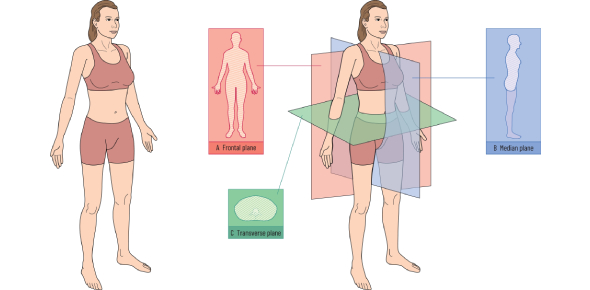# Planes And Axis Of Movement Quiz

11 Questions | Attempts: 9845
ShareSettingsDo you understand the motion of a plane? Take this planes and axis of movement quiz to see how much you know and understand the movement of planes and axis. This is a perfect quiz to practice your knowledge of motion just in a few minutes. All the best! Try for a perfect score on this physics quiz. You can try retaking this quiz even if you miss out on any questions. Do share the quiz with others.

• 1.
The plane which divides the body into superior and inferior halves is known as the ...
• A.

Sagittal plane

• B.

Transverse plane

• C.

Coronal plane

• D.

Frontal plane

• 2.
The plane which divides the body into left and right sides is known as the ...
• A.

Sagittal plane

• B.

Transverse plane

• C.

Horizontal plane

• D.

Coronal plane

• 3.
The plane which divides the body into anterior and posterior halves is known as the ...
• A.

Sagittal plane

• B.

Transverse plane

• C.

Frontal plane

• D.

Horizontal plane

• 4.
The plane which is also known as the coronal plane is ...
• A.

Sagittal plane

• B.

Frontal plane

• C.

Transverse plane

• D.

Horizontal plane

• 5.
The plane which is also known as the horizontal plane is ...
• A.

Transverse plane

• B.

Frontal plane

• C.

Sagittal plane

• D.

None of the above

• 6.
Tiger Woods is primarily moving in the sagittal plane
• A.

True

• B.

False

• 7.
From this view point Tiger is primarily moving in the .....
• A.

Frontal plane

• B.

Sagittal plane

• C.

Transverse plane

• D.

Coronal plane

• 8.
The movement from this view is through the transverse plane
• A.

True

• B.

False

• 9.
Which axis of rotation is formed through the intersection of the sagittal and frontal planes?
• A.

Frontal axis of rotation

• B.

Transverse axis of rotation

• C.

Sagittal axis of rotation

• D.

None of the above

• 10.
The hip flexion occurs as a result of the frontal axis of rotation.
• A.

True

• B.

False

• 11.
The rotation of the upper body around the vertebral column occurs through the vertical axis of rotation. This axis is an intersection of the ___________ and ___________ planes
• A.

Sagittal and frontal

• B.

Sagittal and transverse

• C.

Coronal and transverse

• D.

Frontal and transverse

## Related TopicsBack to top
×

Wait!
Here's an interesting quiz for you.